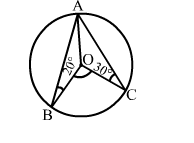# In the given figure, O is the centre of a circle in which ∠OBA = 20° and ∠OCA = 30°.

Question:

In the given figure, O is the centre of a circle in which OBA = 20° and ∠OCA = 30°. Then, ∠BOC = ?
(a) 50°

(b) 90°
(c) 100°
(d) 130°Solution:

(c) 100°
In Δ OAB, we have:
OA = OB          (Radii of a circle)
⇒ ∠OAB = ∠OBA = 20°
In ΔOAC, we have:
OA = OC         (Radii of a circle)
⇒ ∠OAC = ∠OCA = 30°
Now, ∠BAC = (20° + 30°) = 50°
∴ ∠BOC = (2 × ∠BAC) = (2 × 50°) = 100°